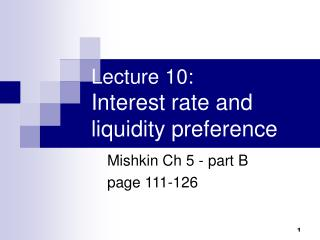DownloadDownload PresentationLecture 10: Interest rate and liquidity preference

# Lecture 10: Interest rate and liquidity preference

Télécharger la présentation## Lecture 10: Interest rate and liquidity preference

- - - - - - - - - - - - - - - - - - - - - - - - - - - E N D - - - - - - - - - - - - - - - - - - - - - - - - - - -
##### Presentation Transcript

1. Lecture 10: Interest rate and liquidity preference Mishkin Ch 5 - part B page 111-126

2. Review • Theory of asset demand • interest rate  bond price, negatively related • move along D&S curves vs. shift of D&S curves • determination: intersection of demand curve (slope downwards) and supply curve (slope upwards) • changes: shifts of demand and supply curves  changes in equilibrium price (i.e. interest rate)

3. Movements along D&S curves • If price is \$950, aggregate demand would be 100 b bonds. • If price is \$850, aggregate demand would be 300 b bonds.

4. Shift of D&S curves • In expansion, wealth increase, for any given price, everyone demands more bonds. back

5. Shift of demand curve (money lender, bond buyer) reasons • expansion • expected inflation decrease; expect future interest rate to be lower than previously anticipated . increase • wealth • expected return • risk • liquidity shift • right • right • left • right • e, real expected return (= nominal return – expected inflation) • Expect i  in future, Pt+1, rate of return for long-term bonds 

6. Shift of supply curve (money borrower, bond seller) increase • profitability of investment • expected inflation • deficit shift • right • right • right • expected inflation e • real interest rate (= nominal interest rate - e ) • real cost of borrowing 

7. Example: expected inflation • real expected return (= nominal return – expected inflation)  D  • real interest rate (= nominal interest rate - e )   S  ‘Fisher Effect’: expected inflation   bond price   interest rate 

8. eand i move together

9. Background • Liquidity preference framework • proposed by John Maynard Keynes. • from the perspective of ‘money’ • assume there are 2 assets: • bond + money = total wealth

10. Bridge • supply side: Ms + Bs = Wealth • demand side: Bd + Md = Wealth  Ms + Bs = Bd + Md subtracting Md and Bs from both sides:  Ms – Md = Bd – Bs Conclusion: If money market is in equilibrium (money demand equals money supply: Md = Ms ), then bond market is also in equilibrium (bond demand equals bond supply: Bd = Bs).

11. Demand curve of money • assume that money earns no interest • as i, relative rate of return on money  (equivalently, opportunity cost of money) Md • Y-axis is now for interest rate ( not Pbond! ) • demand curve (relationship between interest rate and quantity of money demand) slopes downwards

12. Supply curve of money • assume that central bank controls Ms and it is a fixed amount. • supply curve is vertical line.

13. Market equilibrium • market equilibrium: • equilibrium quantity of money: Md = Ms , • ‘price’ of money/equilibrium interest rate: i* • If i > i* , Ms > Md (excess supply of money! central bank supply more money than people are willing to hold!)  price of bonds , i back to i* • If i < i*, Md > Ms (excess demand)  price of bonds , i back to i*

14. Shifts in the demand for money • Income effect: a higher level of income causes the demand for money at each interest rate to increase and the demand curve to shift to the right. • Price-level effect: a rise in the price level causes the demand for money at each interest rate to increase and the demand curve to shift to the right.

15. Shifts in the supply of money • Assume that the supply of money is controlled by the central bank. • An increase in the money supply engineered by the Federal Reserve will shift the supply curve for money to the right.

16. Dose everything else remain equal? • Liquidity preference framework says that an increase in the money supply will lower interest rates, if other things remain unchanged - the liquidity effect. • Noble prize winner Milton Friedman argue: • Income effect finds interest rates rising because increasing the money supply is an expansionary influence on the economy. Ms, expansionary influence on economy  national income , Md, Md shifts right, i

17. Everything else remaining equal? • Price-level effect predicts an increase in the money supply leads to a rise in interest rates in response to the rise in the price level. Ms, price level , Md, Md shifts right, i • Expected-inflation effect shows an increase in interest rates because an increase in the money supply may lead people to expect a higher price level in the future. Ms, e, Bd, Bs, Fisher Effect, i

18. The million-dollar question: If money supply Ms 1. liquidity effect: i 2. income effect: i 3. price-level effect: i 4. expected inflation effect: i What’s the net effect on interest rate? Effect of higher rate of money growth on interest rates is ambiguous.

19. Money Growth & Interest Rates

20. Recap • D&S curves of money and the equilibrium • shifts in D&S curves • effects of money growth on interest rate